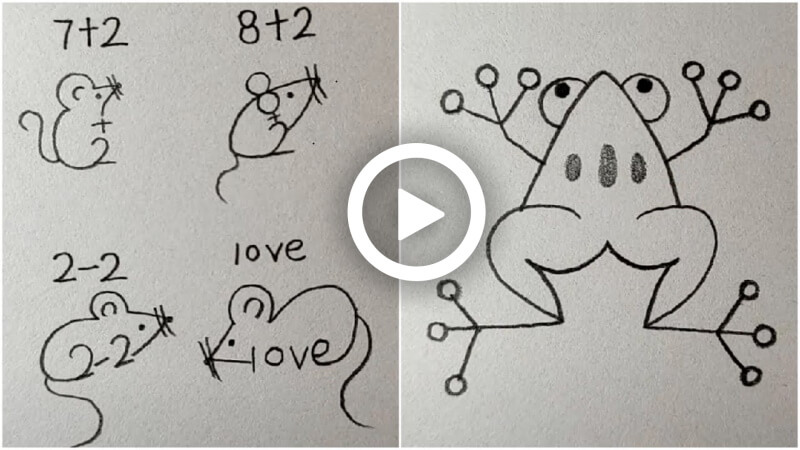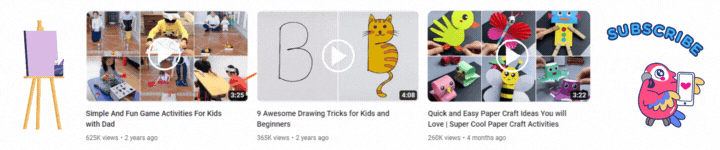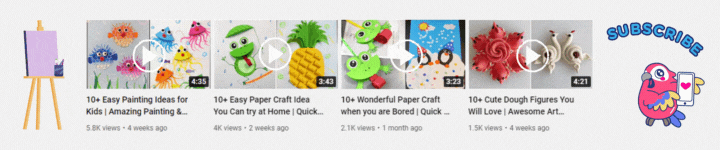Connect with us

#### Easy Animal Drawing for Kids# Easy Animal Drawing for KidsThese are 12 easy and simple drawings you must help your kids with. These amazing drawings will enhance your kids drawing skill as well as knowledge. They will surely enjoy while drawing, so help your kids in drawing these. Down below are the step by step process of drawing these animals, do follow them and draw these beautiful drawings.

## Easy Animal Drawing for Kids

Materials required:

• Paper
• Pencil

### Drawing 1: Bird

Steps:

• Draw a small 3 at the first then a big 3 at the back of it.
• Then from that big 3 draw another curvy 3 merging the top and end with the other 3.
• Now from the small 3 draw a curve on each side and merge it with the big 3 and draw few lines inside it.
• Then inside the two big 3’s draw 3 lines on the top and the bottom.
• Now draw a half oval at the backside of the curvy 3 and inside that draw a circle.
• Then outside the half oval draw a triangle and a line between it as beak.

### Drawing 2: Honey bee

Steps:

• Draw a circle and 2 curvy lines at the top as antenna’s.
• Now draw 2 small lines inside the circle and below that draw 2 circles as eyes.
• Then below the eyes draw a semi oval and a tilted 3 and lines at the ends.
• Now below the circle draw 2 curves and narrow them at the end.
• Then inside it draw few zigzag lines and outside it draw 3 lines on each side and circles on the top of the line.
• Now draw 2 tilted U’s on each side and then from the first small line draw a straight line and draw a star on the top.

### Drawing 3: Frog

Steps:

• Draw a reverse 2 and then leave some space and draw a normal 2.
• Then at the top of both 2’s draw a curvy triangle and at the sides draw 2 semi circles and circle inside each.
• Now from each side of the triangle draw a straight line and then bend the line and draw 2 more lines from the side.
• Then draw circles on the top of the lines and then draw 3 ovals inside the triangle.
• Now in between the two 2’s draw a tilted 3 with less curves on the sides as shown.
• Then from the sides draw a curve and merge them with the 2’s.
• Now from the line of the 2’s draw 2 more lines from the side and then draw circles on the top of the lines.

### Drawing 4: Elephant

Steps:

• Draw a 6 and the leave some space and draw a reverse 6.
• Then below them draw a bigger 6 and reverse 6.
• Now from the middle of both the 6 draw 2 lines and curve them and merge them at the end.
• Then from the line draw a circle and merge it to another line.
• Now draw a semi circle on each side of the circle and then again draw a small semi circle inside it.
• Then at the top of the circle draw a zigzag pattern and then on the inside of the circle draw 2 lines and 2 ovals below that.

### Drawing 5: Hen

Steps:

• Draw a 5 without drawing the upper line of it and then from the straight line draw a triangle shape and a line from middle.
• Now from the top draw a curve and merge it to the end of the 5.
• Then at the top draw 3 reverse U shapes and inside the 5 draw a circle as eye.
• Now draw a leaf pattern inside the 5 and draw 3 lines inside the leaf.
• Then from the end of the 5 draw 2 curves and merge them at the end, do it 3 times more as shown.
• Now at the bottom draw 2 straight lines and then draw again 2 lines on that lines.

### Drawing 6: Cat

Steps:

• Draw 500 and 2 small lines on each 0.
• Then draw a circle from the first 0 to the second 0.
• Now at the top of the circle draw 2 curvy triangles and a curvy line from the middle.
• Then inside the circle draw 2 small lines and then 2 curves below that with few lines on them.
• Now below it draw an oval and then a tilted 3 with few dots.
• Then from the sides of the circle draw 3 lines on each side.
• Now from the curvy triangle draw a curve and merge it with the end of the 5.
• Then draw a line in between the 5 and 0.
• Now from outside of the curve draw 2 curvy lines and merge them together.

### Drawing 7: Dog

Steps:

• Draw 6 0’s and then from the 1st 0 draw a reverse U shape till the 3rd 0.
• Now draw 2 leaf shapes as ears on each side of the reverse U.
• Then inside the U draw 2 lines and below that draw 2 ovals as eyes.
• Now draw an oval between the 2 and 3 0 and draw 3 dots inside the 2 and 3 0.
• Then draw 2 lines on the 1st, 4th, 5th and 6th 0.
• Now draw a curve from the reverse U to the 6th 0.
• Then at the back of the curve draw 2 curvy lines and merge them at the end.
• Now below the 2 and 3 o draw 2 curves and then leaving some space draw a heart and 2 lines below it and at the end again draw a heart.

### Drawing 8: Monkey

Steps:

• Draw a 3 and then at the backside of it draw a small 3 again.
• Then from the first 3 draw another big 3 at the back of it.
• Now at the front of the first 3 draw a reverse 3.
• Then in the front of the bigger 3 draw a curve then again a curve and then a thin U shape merging with the 3.
• Now at the side of the U draw a curve.
• Then inside the reverse 3 draw a circle and then below that a dot and a curve.
• Now below the 3 draw a curvy line and then at the ends draw 2 curves and then again a curvy line.
• Then at the end of the curvy line draw a leaf structure and a line from middle.
• Now at the side of it draw a small leaf.
• Then at the back of the bigger 3 draw a curvy line.

### Drawing 9: Duck

Steps:

• Draw a 2 and at the end extend the line and curve it.
• Now from the front of the 2 draw a curve.
• Then on the front of the 2 draw a line and then curve it a little and again draw a straight line.
• Now again curve a little and draw a straight line.
• Then inside the 2 draw a small line and below that draw a circle.
• Now from the end of the 2 draw few curves one after the other.
• Then again from the end of 2 draw a zigzag curvy structure.
• Now at the bottom draw a wavy pattern.
• Then above the 2 draw a small 2 and from the front of it draw a curve line.
• Now on the front of it draw a line and then curve it a little and again draw a straight line.
• Then again curve a little and draw a straight line.
• Now inside the 2 draw a curve and then from the end of the 2 draw few curves one after the other.
• Then again from the end of 2 draw a 3.

### Drawing 10: Bunny

Steps:

• Draw a circle and then draw a line.
• Then draw a 2 below the circle and then on the top of the circle draw 2 reverse U shapes.
• Now inside the circle draw 2 lines and below that 2 curves with lines on them.
• Then draw a circle and a line and then a curve.
• Now below the curve draw a box with a line in the middle.
• Then from the below of the circle draw a curve inside that 2 lines till the 2.
• Now from the back of the 2 draw a curve inside that 2 lines.
• Then draw a curve below the circle and merge it with the 2 and on the back of it draw a leaf shape as tail.
• Now from the side of the circle draw a curve and merge it with the line.
• Then at the bottom of the line draw an U shape.
• Now at the top of the line draw 3 thin reverse U shapes and a line from middle of each U.

### Drawing 11: Birds

Steps:

• Draw a 2 and then draw another 2 at the back of it.
• Now draw another two 2’s at the back as before and draw a half oval in the middle of the first pair of 2’s.
• Then inside that oval draw a small line and a curve with few small lines on it.
• Now draw a triangle shape outside the oval and a line between the triangle.
• Then draw 2 hearts at the top of it and draw another half oval in the middle of the other pair of 2’s.
• Now inside the oval draw a small line and below that draw a circle as eye.
• Then draw a triangle shape outside the oval and a line in between the triangle.
• Now draw a Question mark and a Exclamation mark at the top of the oval.
• Then from the first 2 draw a curve from the starting point and merge it to the end.
• Now do this with the other pair of 2 as well and draw few lines in the inside of each 2.
• Then draw 3 lines each on the bottom of all 4 2’s and draw 2 horizontal lines.
• Now at the bottom of the first pair draw a line and curve it and draw 2 more curves and draw another line.
• Do the same with the other pair also.

### Drawing 12: Mouse

Step 1: 7+2

• Write down 7+2 in a vertical line and then draw 2 lines on the backside of the 7 with a dot.
• Then from the 7 draw a reverse 3 and inside the 3 draw a curve and inside the 7 draw a dot.
• Now from the backside of the 3 draw a curvy line.

Step 2: 8+2

• Write 8+2 in a vertical line and then from the middle of the 8 draw a triangle shape and at the top draw 2 lines.
• Then inside it draw a dot and from the other side of 8 draw a curve and merge it with 2.
• Now from the backside of the curve draw a curvy line.

Step 3: 2-2

• Write 2-2 in a tilted horizontal line and then draw a greater than symbol and 2 lines at the end with a dot.
• Then draw a dot inside it and then draw a tilted C and a curve till 2.
• Now inside the tilted C draw a small curve.
• Then on the backside of the curve draw a curvy line.

Step 4: love

• Write love in a horizontal line and then on the side of l draw a smaller than symbol with a dot and 2 lines on the tip.
• Then inside it draw a dot and then from the smaller than symbol draw a tilted 3 and inside the 3 draw a curve.
• Now from the back of the 3 draw a curvy line.

That’s it! your 12 different animal drawings are ready. You must try with your kids and help them in drawing these and have fun while drawing. These drawings are super easy and they will enjoy it while drawing, so definitely try these with your kids and have fun.You may also like...
Click to comment